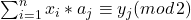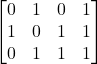# Decoding of Linear Codes

I think I can get through the rest of the appendix this semester.  Fingers crossed.

The problem: Decoding of Linear Codes.  This is problem MS7 in the appendix.

The description: We are given an NxM boolean matrix A, a vector y with m 0’s and 1’s in it, and an integer K.  Does there exist a boolean vector x, with n elements, and at most K 1’s, where for each column j:?

Example: Here is a matrix A:If our vector y is (1,0,0,1), we are saying we want a vector x that is different from the first and fourth column of A in one (or 3) positions and is different from the second and third column in 0 (or 2) positions.   A vector such as (0,1,1) will do this.

If y was (1,1,1,1), I don’t think it’s possible to come up with any vector x, because since the first two columns of A are opposites, any vector that differs in the first column in 1 (or 3) positions will differ in the second column in 2 (or 0) positions, so we won’t be able to satisfy both elements of y simultaneously.

Reduction:

The paper by Berlekamp, McEliece, and van Tilborg calls this the  “Coset Weights” problem, and uses 3DM, adding the assumption that all 3 dimensions come from the same set of parent elements. So we start with a set U (they call it “W” in the paper) of triples.

We will use these triples to build our matrix A.  Our matrix will have one row for each triple and 3 columns for each element in our parent set (so 3q columns total).  We then build an incidence matrix of each of our triples- each row will have exactly three 1’s in it, corresponding to the element in each of the three dimensions it is.  So, for example, if the sets we are making our triples from were all {1,2,3,4}, and our triple was (1,2,3), the row for that triple would be {1,0,0,0, 0,1,0,0,0,0,1,0}

We’ll choose y to be a vector of all 1’s.  Now we are looking for a vector x of 0’s and 1’s.  This will be like choosing triples from the 3DM instance.  (A 1 in the vector means we choose that row and a 0 means we don’t).  We’ll set K=q to make sure that we choose exactly q triples.

So, a set of q triples that covers all elements will have a 1 in each column in one of these triples and give us a solution to our problem. Similarly, a vector with q 1’s in the correct places that covers all columns will generate our y vector.

Difficulty: 5.  Matrix manipulation is something I personally don’t feel terribly comfortable with, but this is a pretty straightforward implementation of it.